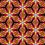# Set theory concern

For a given set, let's say $$A$$, is it true that $$\varnothing \subset A$$ ?

My personal thought is that it's not true, Since:

Let $A = \{1,2,3,4 \}$, then to say that $\varnothing \subset A$, we say that every element of $\varnothing$ (nothing) also belongs to the set $A$, which is strange to say since $A = \{1,2,3,4\}$.

On the other hand to say that $\varnothing \subset A$, nothing seems to change in the set $A$ since $\varnothing$ has no elements...

Would it be necessary to set a condition such that $A = \varnothing$ ?

What am I missing ? Any thoughts ?Note by André Hucek
3 years, 9 months ago

This discussion board is a place to discuss our Daily Challenges and the math and science related to those challenges. Explanations are more than just a solution — they should explain the steps and thinking strategies that you used to obtain the solution. Comments should further the discussion of math and science.

When posting on Brilliant:

• Use the emojis to react to an explanation, whether you're congratulating a job well done , or just really confused .
• Ask specific questions about the challenge or the steps in somebody's explanation. Well-posed questions can add a lot to the discussion, but posting "I don't understand!" doesn't help anyone.
• Try to contribute something new to the discussion, whether it is an extension, generalization or other idea related to the challenge.

MarkdownAppears as
*italics* or _italics_ italics
**bold** or __bold__ bold
- bulleted- list
• bulleted
• list
1. numbered2. list
1. numbered
2. list
Note: you must add a full line of space before and after lists for them to show up correctly
paragraph 1paragraph 2

paragraph 1

paragraph 2

[example link](https://brilliant.org)example link
> This is a quote
This is a quote
    # I indented these lines
# 4 spaces, and now they show
# up as a code block.

print "hello world"
# I indented these lines
# 4 spaces, and now they show
# up as a code block.

print "hello world"
MathAppears as
Remember to wrap math in $$ ... $$ or $ ... $ to ensure proper formatting.
2 \times 3 $2 \times 3$
2^{34} $2^{34}$
a_{i-1} $a_{i-1}$
\frac{2}{3} $\frac{2}{3}$
\sqrt{2} $\sqrt{2}$
\sum_{i=1}^3 $\sum_{i=1}^3$
\sin \theta $\sin \theta$
\boxed{123} $\boxed{123}$

Sort by:

The empty set is indeed contained in any other set. The condition that every element of the empty set is contained in $A$ is always satisfied, because the empty set doesn't have any elements. So there is no restriction.

- 3 years, 9 months ago

I later thought so, thank you for your answer, though i wonder if it can be proven !

- 3 years, 9 months ago

It is true that $\emptyset \subset A$ for all sets.

It is not true that "$\emptyset$ is nothing". It is the empty set.

See Sets - Subsets.

Staff - 3 years, 9 months ago

"Nothing" wasn't to the set, but the elements of it, I will correct it

- 3 years, 9 months ago

Great, so now you have the right statement. Since there are no elements in the set, it is true that all elements of the empty set are in A.

The empty set can sometimes be strange. A lot of statements about it are true, even seemingly contradictory ones. For example, "All the mini coopers I own are red" and "All the mini coopers I own are blue" are both true statements, because the empty set (mini coopers I own) belong to every other set out there.

Staff - 3 years, 9 months ago

The empty set is sometimes confusing, one has to be more careful how to use the english in mathematics the correct way.

- 3 years, 9 months ago